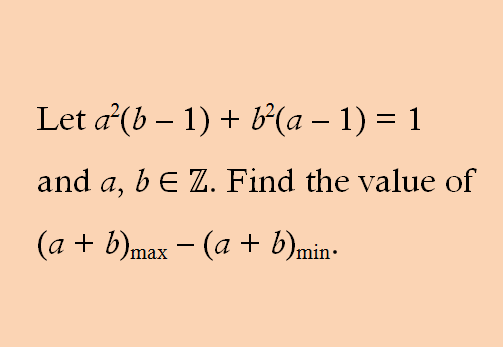# Week 2

Let a2(b ⎼ 1) + b2(a ⎼ 1) = 1 and a, b ∈ ℤ. Find the value of (a + b)max – (a + b)min.

Let x = b – 1 and y = a – 1, so that x, y ∈ ℤ.

Given equation takes the form

(y + 1)2x + (x + 1)2y = 1

Or, (y2 + 2y + 1)x + (x2 + 2x + 1)y = 1

Or, y2x + 2xy + x + x2y + 2xy + y = 1

Or, y2x + x2y + 2xy + 2xy + x + y = 1

Or, xy(x + y + 4) + 1(x + y + 4) = 1 + 4 = 5

Or, (x + y + 4)(xy + 1) = 1 × 5, 5 × 1, ⎼1 × ⎼5, ⎼5 × ⎼1.

Case I. Let x + y + 4 = 5 and xy + 1 = 1.

Then (x, y) = (1, 0), (0, 1), so that (a, b) = (2, 1), (1, 2).

Case II. Let x + y + 4 = 1 and xy + 1 = 5.

Since x, y ∈ ℤ, this is not possible.

Case III. Let x + y + 4 = ⎼1 and xy + 1 = ⎼5.

Then (x, y) = (⎼6, 1), (1, ⎼6), so that (a, b) = (⎼5, 2), (2, ⎼5).

Case IV. Let x + y + 4 = ⎼5 and xy + 1 = ⎼1.

Since x, y ∈ ℤ, this is not possible.

Hence, (a + b)max = 3 and (a + b)min = ⎼3.

Therefore, (a + b)max – (a + b)min = 3 ⎼ (⎼3) = 6.